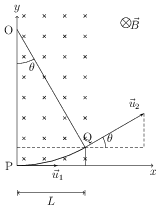# Force on a Moving Charge in a Uniform Magnetic Field

## Problems from IIT JEE

Problem (IIT JEE 2013): A particle of mass $M$ and positive charge $Q$, moving with a constant velocity $\vec{u}_1={4\hat{\imath}}\;\mathrm{m/s}$, enters a region of uniform static magnetic field normal to the $x\text{-}y$ plane. The region of the magnetic field extends from $x=0$ to $x=L$ for all values of $y$. After passing through this region, the particle emerges on the other side after $10$ milliseconds with a velocity $\vec{u}_2={2\left(\sqrt{3}\hat{\imath}+\hat{\jmath}\right)}\;\mathrm{m/s}$. The correct statement(s) is (are)

1. The direction of the magnetic field is $-z$ direction.
2. The direction of the magnetic field is $+z$ direction.
3. The magnitude of the magnetic field is $\frac{50\pi M}{3Q}$ units.
4. The magnitude of the magnetic field is $\frac{100\pi M}{3Q}$ units.

Solution:The path followed by the particle is a circular arc PQ with $\vec{u}_1$ and $\vec{u}_2$ tangential at P and Q (see figure). The $\vec{u}_2=2(\sqrt{3}\,\hat{\imath}+\hat{\jmath})$ gives $\theta=\pi/6$. For this motion, magnetic field ($\vec{B}$) shall be in $-z$ direction so that $Q\,\vec{v}\times\vec{B}$ provide necessary centripetal acceleration. From Newton's second law, $QvB=\frac{Mv^2}{r}$, which gives $\omega=\frac{v}{r}=\frac{QB}{M}$, where $v$ is velocity, $\omega$ is angular velocity and $r$ is radius of the circular path. The angular displacement in time $t$ is $\theta=\omega t=\frac{QB}{M}t$. Substituting for $\theta$ and $t$, we get, $B=\frac{50}{3}\frac{\pi M}{Q}$.